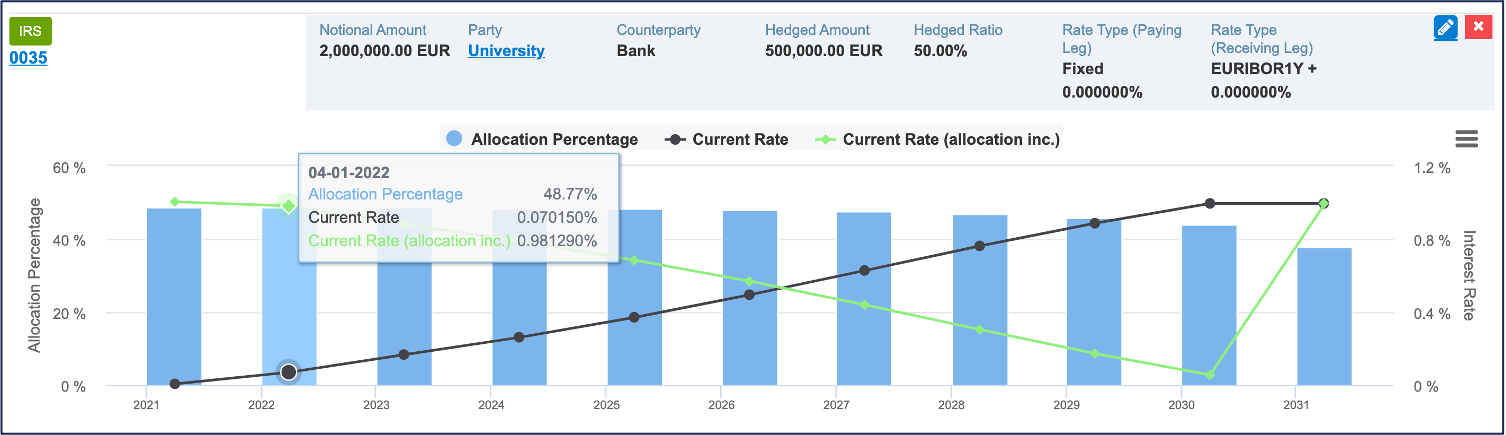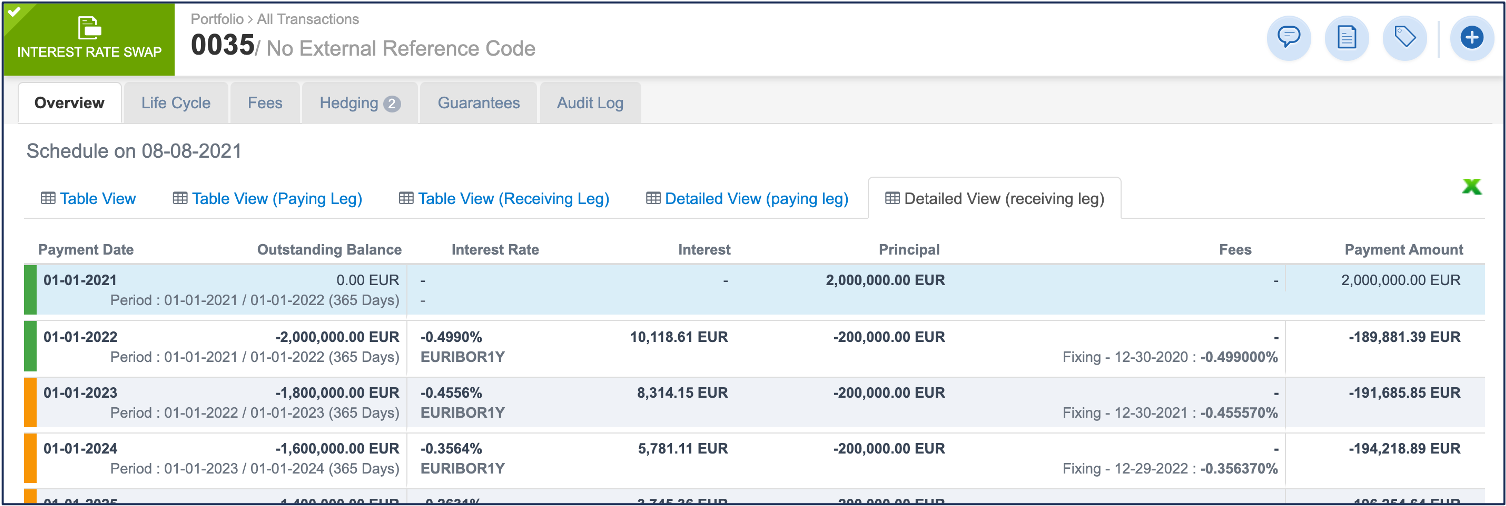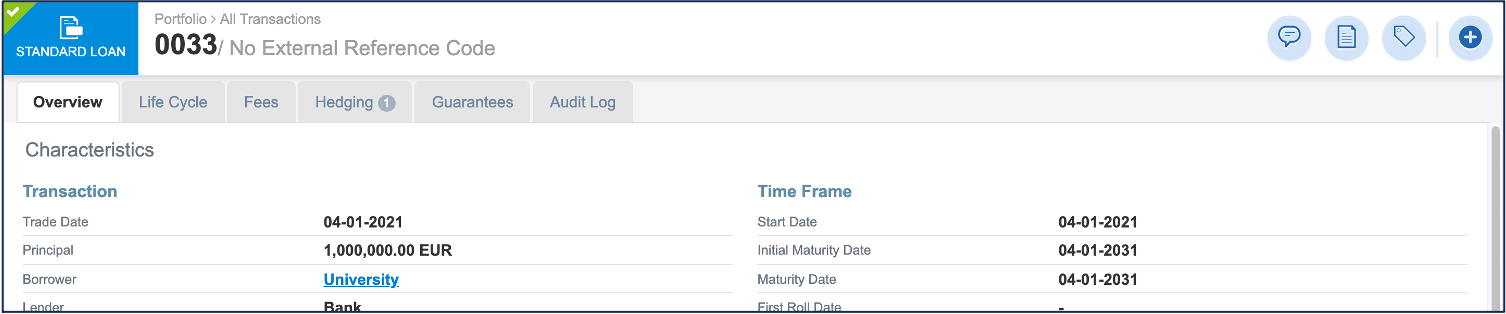# Transaction Hedging Overview

Hedging in Fairways Debt presents the relationship of the actual debt and the risk hedging transactions.

1. Navigate to Applications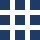> Debt & Derivatives.
2. Click a hedged transaction to open its profile.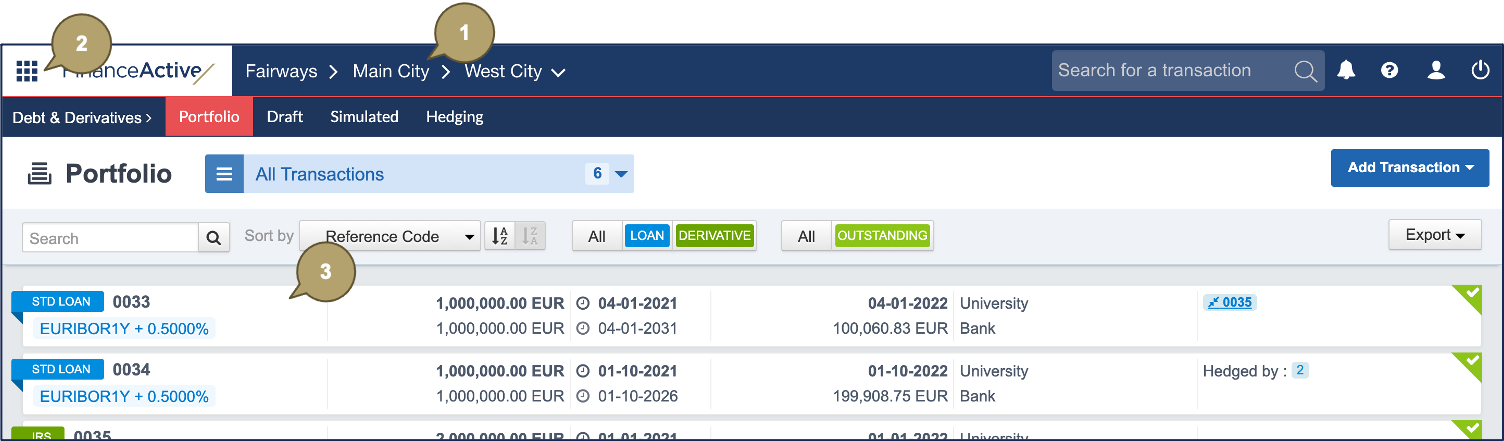1. Navigate to Hedging.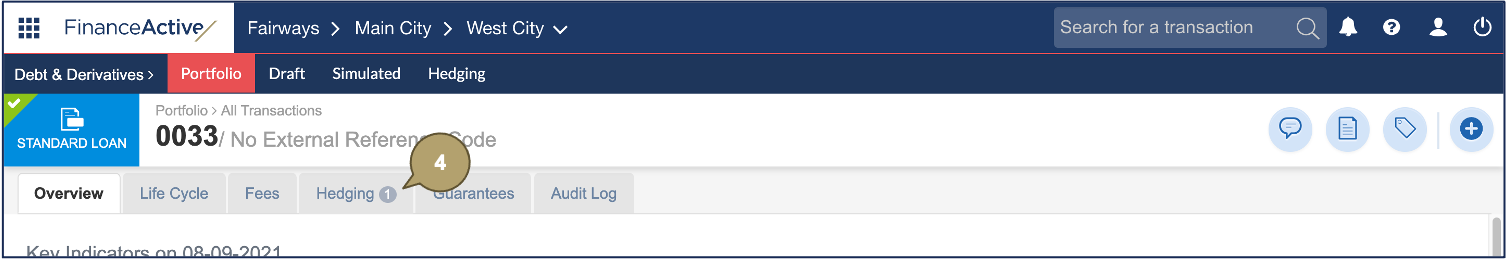The hedging overview displays.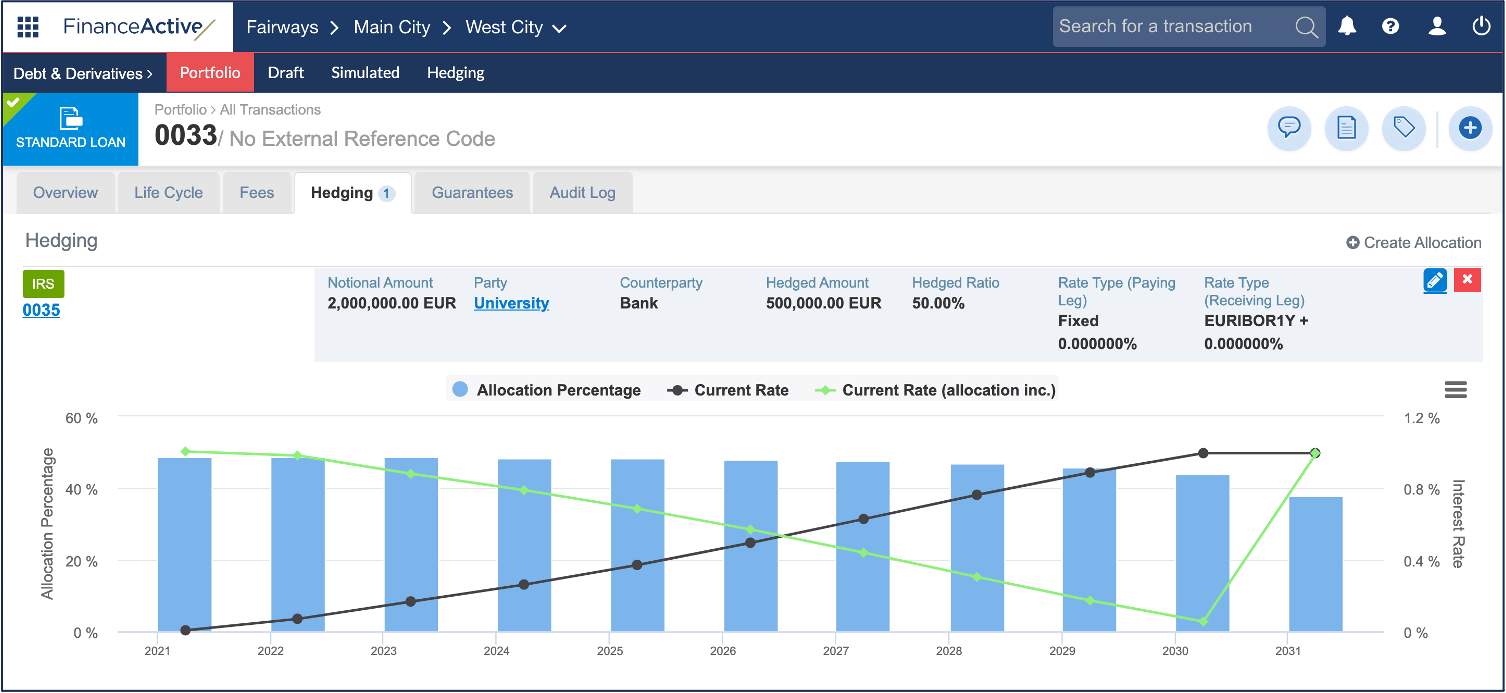Indicator

Description

Current Rate

Current interest rate of the hedged transaction.

Current Rate (allocation inc.)

[Notional Amount * (Current Rate + Interest Rate (Paid) - Interest Rate (Received)) + Current Rate * (Principal - Notional Amount)] / Principal

In this example, the current rate including the allocation on 1 April 2022 is 0,98129%.

[Notional Amount * (Current Rate + Interest Rate (Paid) - Interest Rate (Received)) + Current Rate * (Principal - Notional Amount)] / Principal

= [2 000 000 * (0,070 150 + 0 - (-0,45557)) + 0,070 150 * (1 000 000 - 2 000 000)] / 1 000 000

=0,98129%